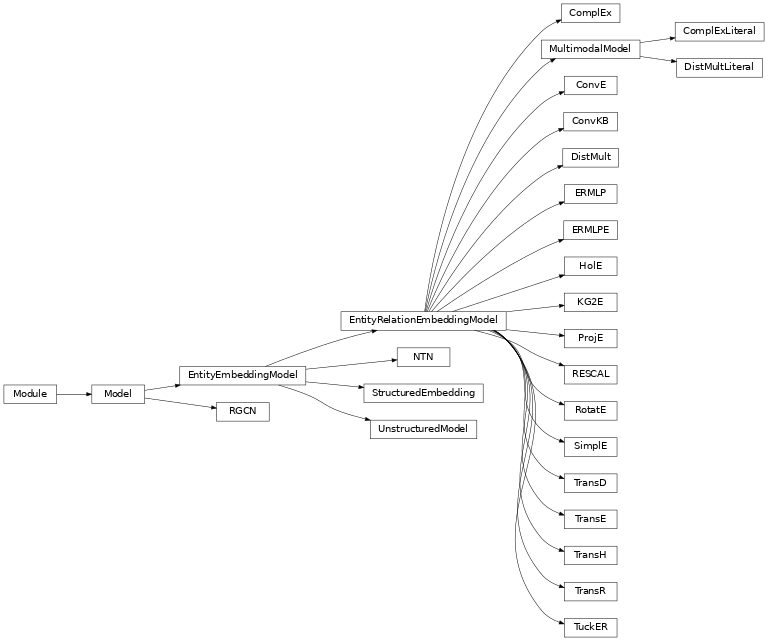# Models¶

An interaction model $$f:\mathcal{E} \times \mathcal{R} \times \mathcal{E} \rightarrow \mathbb{R}$$ computes a real-valued score representing the plausibility of a triple $$(h,r,t) \in \mathbb{K}$$ given the embeddings for the entities and relations. In general, a larger score indicates a higher plausibility. The interpretation of the score value is model-dependent, and usually it cannot be directly interpreted as a probability.

## Functions¶

 get_model_cls(query) Look up a model class by name (case/punctuation insensitive) in pykeen.models.models.

## Classes¶

 ComplEx(triples_factory[, embedding_dim, …]) An implementation of ComplEx [trouillon2016]. ComplExLiteral(triples_factory[, …]) An implementation of ComplexLiteral from [agustinus2018] based on the LCWA training approach. ConvE(triples_factory[, input_channels, …]) An implementation of ConvE from [dettmers2018]. ConvKB(triples_factory[, …]) An implementation of ConvKB from [nguyen2018]. DistMult(triples_factory[, embedding_dim, …]) An implementation of DistMult from [yang2014]. DistMultLiteral(triples_factory[, …]) An implementation of DistMultLiteral from [agustinus2018]. ERMLP(triples_factory[, embedding_dim, …]) An implementation of ERMLP from [dong2014]. ERMLPE(triples_factory[, hidden_dim, …]) An extension of ERMLP proposed by [sharifzadeh2019]. HolE(triples_factory[, embedding_dim, …]) An implementation of HolE [nickel2016]. KG2E(triples_factory[, embedding_dim, …]) An implementation of KG2E from [he2015]. NTN(triples_factory[, embedding_dim, …]) An implementation of NTN from [socher2013]. ProjE(triples_factory[, embedding_dim, …]) An implementation of ProjE from [shi2017]. RESCAL(triples_factory[, embedding_dim, …]) An implementation of RESCAL from [nickel2011]. RGCN(triples_factory[, embedding_dim, …]) An implementation of R-GCN from [schlichtkrull2018]. RotatE(triples_factory[, embedding_dim, …]) An implementation of RotatE from [sun2019]. SimplE(triples_factory[, embedding_dim, …]) An implementation of SimplE [kazemi2018]. StructuredEmbedding(triples_factory[, …]) An implementation of the Structured Embedding (SE) published by [bordes2011]. TransD(triples_factory[, embedding_dim, …]) An implementation of TransD from [ji2015]. TransE(triples_factory[, embedding_dim, …]) TransE models relations as a translation from head to tail entities in $$\textbf{e}$$ [bordes2013]. TransH(triples_factory[, embedding_dim, …]) An implementation of TransH [wang2014]. TransR(triples_factory[, embedding_dim, …]) An implementation of TransR from [lin2015]. TuckER(triples_factory[, embedding_dim, …]) An implementation of TuckEr from [balazevic2019]. UnstructuredModel(triples_factory[, …]) An implementation of the Unstructured Model (UM) published by [bordes2014].

## Class Inheritance Diagram¶## Base Classes¶

Base module for all KGE models.

### Classes¶

 Model(triples_factory[, loss, …]) A base module for all of the KGE models. EntityEmbeddingModel(triples_factory[, …]) A base module for most KGE models that have one embedding for entities. EntityRelationEmbeddingModel(triples_factory) A base module for KGE models that have different embeddings for entities and relations. MultimodalModel(triples_factory[, …]) A multimodal KGE model.

## Initialization¶

Embedding weight initialization routines.

embedding_xavier_normal_(embedding, gain=1.0)[source]

Initialize weights of embedding similarly to Glorot/Xavier initialization.

Parameters
• embedding (Embedding) – An embedding

• gain (float) – An optional scaling factor, defaults to 1.0.

Return type

Embedding

Returns

Embedding with weights by the Xavier normal initializer.

Proceed as if it was a linear layer with fan_in of zero and Xavier normal initialization is used. Fill the weight of input embedding with values values sampled from $$\mathcal{N}(0, a^2)$$ where

$a = \text{gain} \times \sqrt{\frac{2}{\text{embedding_dim}}}$

In the following example, an embedding is initialized using the suggested gain for the rectified linear unit (ReLu).

>>> e = nn.Embedding(num_embeddings=3, embedding_dim=5)
>>> embedding_xavier_normal_(embedding=e, gain=nn.init.calculate_gain('relu'))

embedding_xavier_uniform_(embedding, gain=1.0)[source]

Initialize weights of embedding similarly to Glorot/Xavier initialization.

Proceed as if it was a linear layer with fan_in of zero and Xavier uniform initialization is used, i.e. fill the weight of input embedding with values values sampled from $$\mathcal{U}(-a, a)$$ where

$a = \text{gain} \times \sqrt{\frac{6}{\text{embedding_dim}}}$
Parameters
• embedding (Embedding) – An embedding

• gain (float) – An optional scaling factor, defaults to 1.0.

Return type

Embedding

Returns

Embedding with weights by the Xavier uniform initializer.

In the following example, an embedding is initialized using the suggested gain for the rectified linear unit (ReLu).

>>> e = nn.Embedding(num_embeddings=3, embedding_dim=5)
>>> embedding_xavier_uniform_(embedding=e, gain=nn.init.calculate_gain('relu'))